客服热线：133-4342-757518953131868•••# 济南拉力试验机 拉力试验机

• 面议

CJK-1464

18953131868 053185709838
• 发货地  山东
• 供货总量  66 台

• 暂无产品目录
 有效期至长期有效 更新时间2020-07-11 04:56 访问次数685

rr

r

r 该拉力试验机采用交流伺服电动机和交流伺服调速系统作为动力源；采用先进的芯片集成技术，专业设计的数据采集放大和控制系统，试验力、变形的放大、A/D转换过程实现了控制和显示的全数字化调整。本机可对各种金属、非金属及复合材料进行力学性能测试和分析研究，广泛应用于航空航天、石油化工、机械制造、电线、电缆、纺织、纤维、塑料、橡胶、陶瓷、食品、医药包装、铝塑管、塑料门窗、土工布、薄膜、木材、纸张、金属材料及制造业，可根据GB、JIS、ASTM、DIN、ISO等标准自动求取最大试验力值、断裂力值、屈服强度、上下屈服强度、抗拉强度、抗压强度、断裂延伸率、拉伸弹性模量、弯曲弹性模量等试验数据。  r

rr r r r r r r r r r r r r r r r r r r r r r r r r r r r r r r r r r r r r r r r r r r r r r r r r r r r r r r r r r r r r r r r r r r r r r r r r r r r r r r r r r r r r r r r r r r r r r
 r r 产品型号 r r r r WDW-10 r r r r WDW-20 r r r r WDW-50 r r r r WDW-100 r r r r WDW-200 r r r r WDW-300 r r r r 最大试验力 r r r r 10 r r r r 20 r r r r 50 r r r r 100 r r r r 200 r r r r 300 KN r r r r 试验力 r r r 测量范围 r r r r 最大试验力的2%--100 r r r r 试验力分辨力 r r r r 1/300000(全程不分档或等效6档) r r r r 试验力 r r r 示值精度 r r r r 优于示值±1% r r r r 横梁位移测量 r r r r 分辨率高于0.0025mm r r r r 变形测量精度 r r r r ±0.5% r r r r 试验速度范围 r r r r 0.01—500mm/min,无级调速 r r r r 速度控制精度 r r r r ±1%(0.01~10mm/min); ±0.5%(10~500mm/min) r r r r 恒力、恒变形 r r r 恒位移控制范围 r r r r 设定值<10%FS时,设定值的±1.0%以内 r r r 设定值≥10%FS时,设定值的±0.1%以内 r r r r 变形速率 r r r 控制精度 r r r r 速率<0.05%FS时为±2.0%设定值内 r r r 速率≥0.05%FS时为±0.5%设定值内 r r r r 夹具 r r r r 形式 r r r r 楔形夹具体 r r r r 平钳口 r r r r 0-7mm r r r r 0-14mm r r r r 0-20mm r r r r 圆钳口 r r r r φ4-φ9mm r r r r φ4-φ14mm r r r r φ9-φ26mm r r r r φ9-φ32mm r r r r 最大拉伸空间 r r r r 600mm r r r r 最大压缩空间 r r r r 700mm r r r r 有效试验宽度 r r r r 400mm r r r r 570mm r r r r 整机电源 r r r r 单相,220V±10%,50Hz r r r r 三相,380V±10%,50Hz r r
rr

r 结构与原理及特点 r

r

r WDW系列电子式拉力试验机，由主机、全数字测量控制系统、用户软件包、功能附件等部件组成。 r

r

r  主机 r

r

r 1.1 主机为门式预应力框架，轴向刚度高。它主要由上横梁、移动横梁(中间部位)、台面及光杠组成框架式结构，滚珠丝杠固定在台面和上横梁之间。两滚珠丝杠之丝母及两光杠之导套固定在移动横梁上。 r

r

r 1.2 结构原理： 采用圆弧同步带轮减速，德国霹西公司产滚球丝杠副传动，传动无间隙，使试验力和变形速度精密控制得到保证。电机通过三级同步带轮减速以后带动丝杠旋转，从而推动移动横梁在选定的速度下作直线运动以实现各种试验功能. r

r

r 1.3 采用了双空间结构，上空间用于拉伸试验；下空间做压缩、弯曲试验。 r

r

r 1.4 传动平稳，响应快，噪声低。 r

r

r 1.5 伺服单元采用全数字交流伺服电机和驱动器，调速范围宽，精度高。 r

r

r 1.6 具有自动调零、自动标定等功能； r

r

r 1.7 保护功能：具有超载、超行程、过压、过流、过热等保护功能； r

r

r 1）移动横梁限位保护机构： r

r

r 由限位杆、上下挡块、紧固螺钉、拔叉、限位开关等组成。 r

r

r 为了防止移动横梁超过上、下极限位置造成机械事故或使移动横梁能停在设定位置，试验机设有一个移动横梁限位保护机构。当移动横梁上的拔叉碰到挡块时，便通过限位杆、触片碰压限位开关的触点，从而使试验机停车。 r

r

r 2）“紧急停车”：在您使用试验机过程中出现异常情况，不能以正常手段关机时，请立即按下“紧急停车”按钮，保护试验机不被意外损坏。 r

r

r 1.8 力通道接口可更换其它类型的力传感器，自动标定。变形通道接口亦可更换其它类型的变形传感器，自动标定。 r

r

r 测量控制系统介绍 r

r

r 一）多功能测量控制卡介绍 r

r

r 1. 使用方式   安插在计算机主机内。 r

r

r ① 真正实现与计算机机电一体化，由一台计算机完成全部测量控制过程。 r

r

r ② 打破了以往行业内单片机系统模拟电路，或独立的测控箱与计算机通讯，且环节复杂，故障率高，抗干扰能力弱的特点。 r

r

r   ③ 多通道的全数字数据放大、智能采集，闭环程序控制于一身，集成度高，稳定可靠。 r

r

r ④ 具备控制系统本身自检功能，自我诊断，自动提示故障发生之处，系统维修仅需华衡邮寄更新计算机内的功能测量卡即可，如同安插网卡一样方便，系统自动生成。 r

r

r 2． 适用范围 r

r

r PCI卡集多种模拟传感器放大器、A/D采集、数字传感器采集、PID闭环控制于一体，配有完善的综合管理的windows设备驱动程序和测控软件动态库，是综合完整的测量及控制系统。可控制伺服电机和液压伺服控制的设备。既可单个卡独立使用，亦可多卡并列使用。在有些设备中，需要对多个伺服电机或多个液压伺服系统管理，既可采用多个卡并列使用，完成多个伺服执行单元的管理。 r

r

r 3． 功能组成 r

r

r ①三路模拟传感器宽范围测量通道；测量范围从1%-100，满足1%精度。三路模拟传感器精密宽范围测量功能，每路测量都可以接入不同类型的模拟传感器，如力传感器、压力传感器、电子尺、直流差动变压器、热电偶等传感器，对其直接测量。
r②三路数字传感器测量通道：每路测量都可接入不同类型的数字传感器，如光电编码器、光栅尺等。
r③一路闭环控制通道：可设定任意三路测量通道作为反馈，实现三路闭环控制。速度可连续调整，三种控制随意切换。在可编成控制模式下，可设定实现任意运行过程。并可实现正弦波、三角波、梯形波及任意组合波形的控制。 r

r

r 4． 软件功能 r

r

r 软件按模块化设计，本系统采用软件管理硬件工作的，测量管理和调速控制软件全部采用VC++语言编程。标准的windows设备驱动程序：可安装于windows 2000/XP/NT/98 等操作系统；由四部分组成：
r①模拟测量模块：可对三路电子模拟测量通道进行配置、标定、调零、测试、测量管理；
r②数字测量模块：可对三路电子数字测量通道进行配置、标定、调零、测试、测量管理；    r

r

r ③调速控制模块：可对电子数字控制通道进行配置、测试、控制输出管理。
r④综合管理模块：对上述三个模块管理。同时具有： ⑴对所有模拟测量和数字测量统一编号，编号可根据需要任意改变；硬件综合测试功能；⑵组合波形函数信号发生器功能：可产生斜波、正弦波、三角波、梯形波以及组合波形，供PID控制使用； r

r

r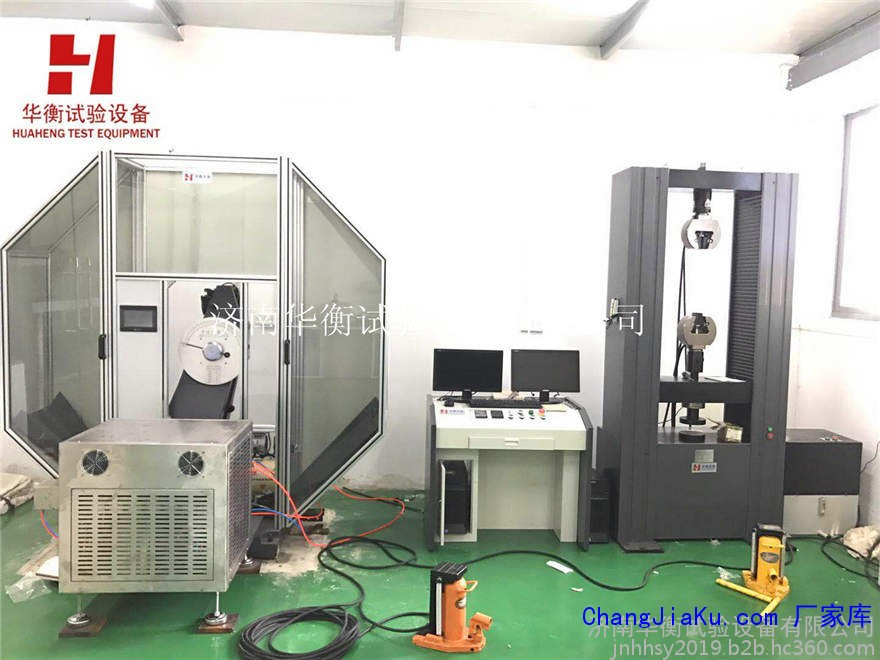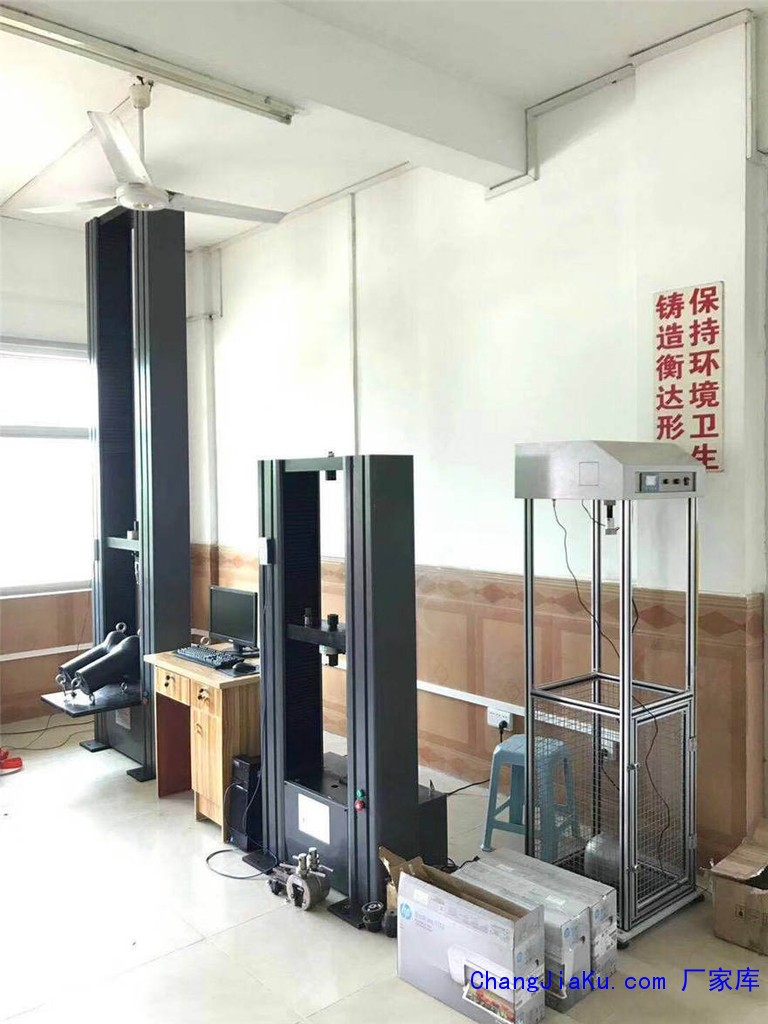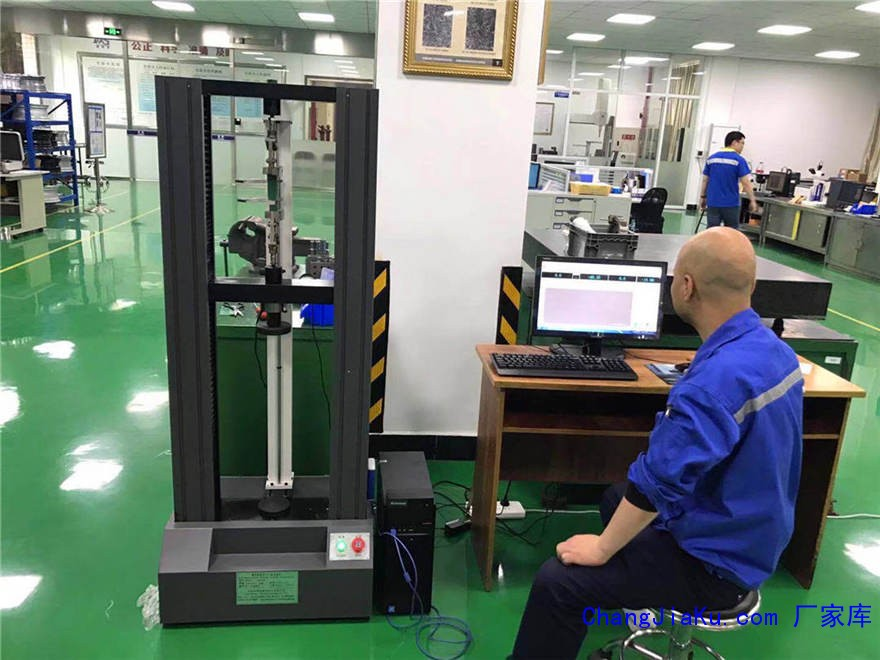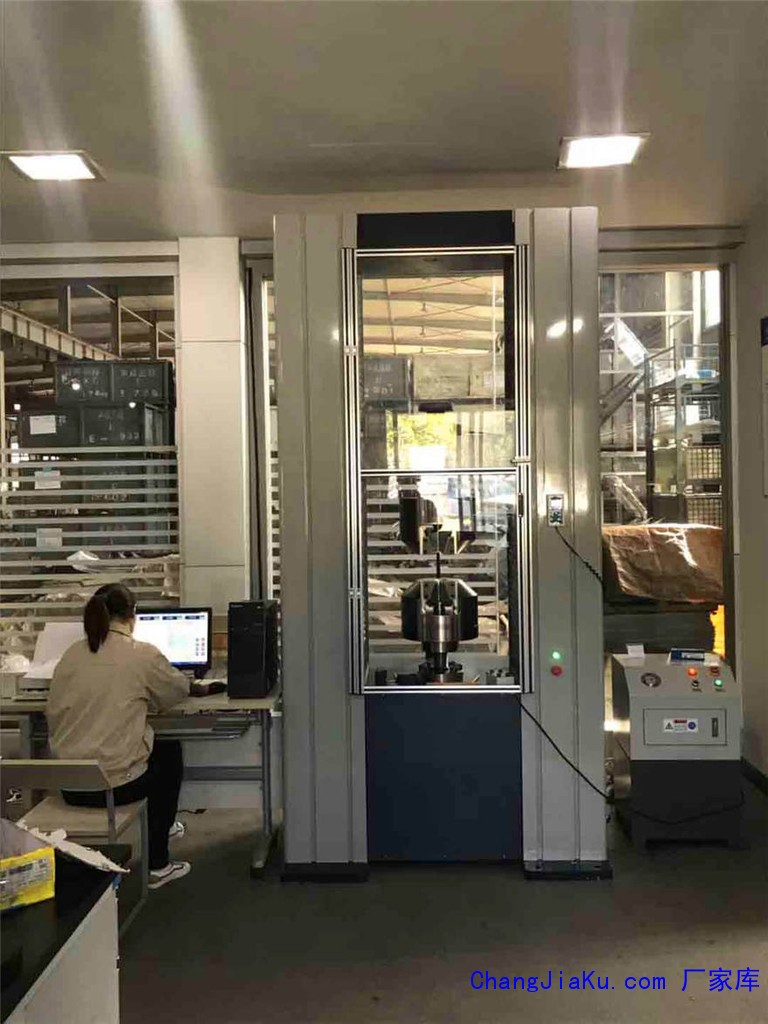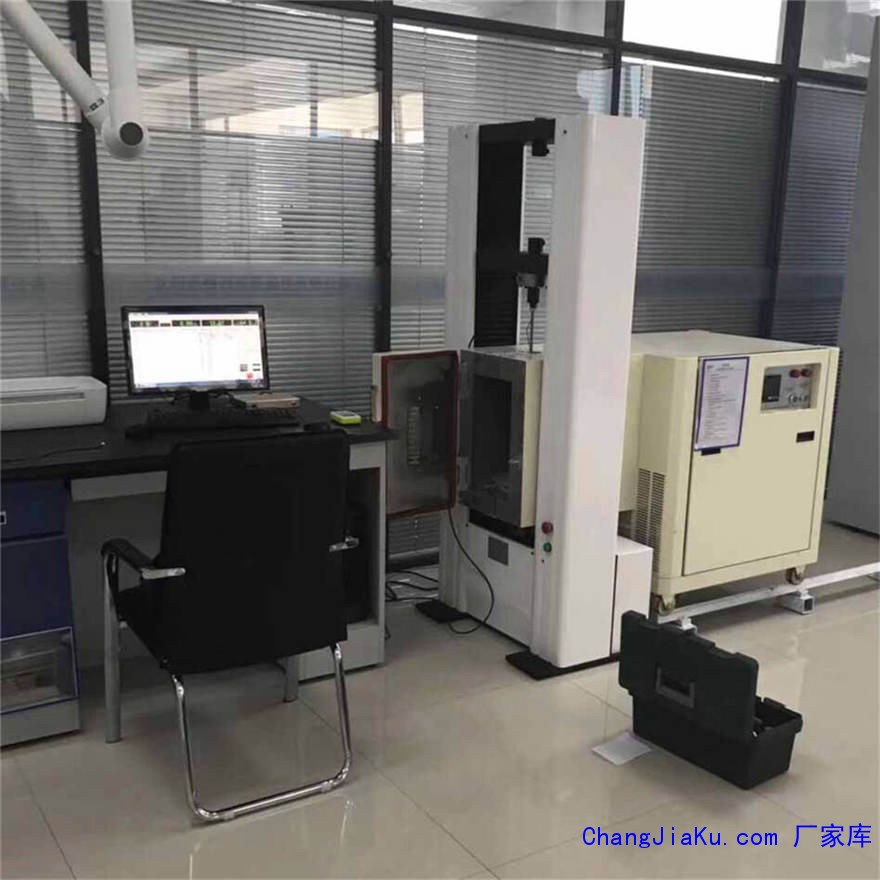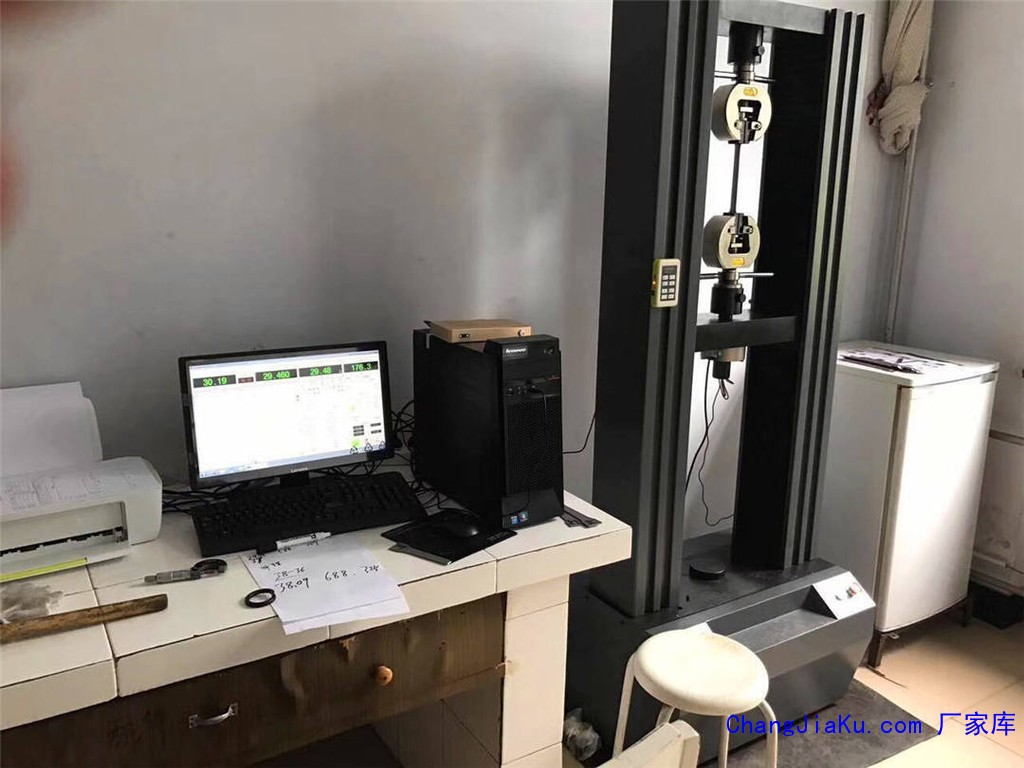r

## 剥离强度试验机,撕裂试验机（90°剥离试验## 往复摩擦磨损试验机 往复摩擦试验机 往复磨r rrrrrrGB3960-2016塑料滑动磨损试验机GB 12444.1-1990 金属磨损试验试验机 rrrr rrr该机适用于SH/T0190-92液体润滑剂摩擦系数测定法，GB12444.1-90金属磨损试验方法及GB3960-2016塑料滑动摩擦磨损试验方法，可做各种金属材料及非金属材料（尼龙、塑料等）在滑动摩擦、滚动摩擦、滚动滑动复合摩擦和间歇接触摩擦各种状态下的耐摩性能试验，并可模拟各种材料在不同的摩擦条件下进行湿摩擦、干摩擦以及磨料磨损等多种试验，可测定各

## 济南弹簧拉压试验机厂家直销,弹簧试验机,弹rrrrrrrrrrr一、参照标准：r 《JB/T 7796-2005 弹簧拉压试验机》本标准规定了弹簧拉压试验机的术语和定义、要求、检验规则、标志和包装等内容。r二、试验对象：r TLW微机控制弹簧拉压试验机适用于拉簧、压簧、碟簧、塔簧、板簧、卡簧、片弹簧、复合弹簧、气弹簧、模具弹簧、异形弹簧等精密弹簧的拉力、压力、位移、刚度等进行试验和分析，并能打印曲线、数据报告。r三、功能特点：r1.拉伸试验：对各种拉伸弹簧做拉伸试验r2.压缩试

## 环刚度压力试验机,波纹管环刚度试验机 管材r rrr rrr rrrrrr电子式拉力机 微机控制电子拉力试验机拉力试验机 智能拉力机 微电脑拉力试验机 rrr一、功能与用途： rrr该试验机采用交流伺服电动机和交流伺服调速系统作为动力源；采用先进的芯片集成技术，专业设计的数据采集放大和控制系统，试验力、变形的放大、A/D转换过程实现了控制和显示的全数字化调整。本机可对各种金属、非金属及复合材料进行力学性能测试和分析研究，广泛应用于航空航天、石油化工、机械制造、电线、电

## 华衡标准件拉力试验机 螺栓保证载荷试验机r rrrrrr微机控制电液伺服拉力试验机 rrr rrr1简介 rrr微机控制电液伺服拉力试验机主机采用油缸下置式主机，采用微机控制和显示功能！主要用于金属、非金属的拉伸、压缩、弯曲和剪切试验。适用于冶金、建筑、轻工、航空、航天、材料、大专院校、科研单位等领域。试验操作和数据处理符合GB228-2002《室温材料 金属拉伸试验方法》的要求。 rrr2 方案描述 rrr2.1 主机 rrr主机采用油缸下置式主机，拉伸空间位于主机的上，压缩、弯曲试

## 拉力试验机 ，拉力试验机厂家，拉力试验机## 摆锤冲击试验机 塑料金属冲击试验机 悬臂梁r rrr rrrJB-300B半自动冲击试验机 rrr一、产品综述 rrr（一）原理rrr本公司生产的JB系列冲击试验机是严格按国标GB/T3808-2007《摆锤式冲击试验机的检验》开发的产品，按国标GB/T229-2007《金属夏比缺口冲击试验方法》对金属材料进行冲击试验，按JJG145-82《摆锤式冲击试验机》出厂检验。所用式样断面为10*10MM。主要对冲击韧性较大的黑色金属，特别是钢铁及其合金进行试验。rrr该产品的试验原理是利用摆锤冲击前位能与冲击后所剩

## 纸箱抗压试验机厂家直销 纸箱压力试验机,包